## LATESTLDC EXAM PREPARATION    ||SI / BDO EXAM PREPARATION 2020    ||PREVIOUS QUESTIONS 2020 DEGREE LEVEL     ||PREVIOUS QUESTIONS LDCPSC EXAM PROGRAMME 2020     ||TODAY'S EXAM RESULTS 2020    ||FINAL ANSWER KEY    ||    FREE STUDY MATERIALS (LDC)     ||    FREE STUDY MATERIALS (DEGREE LEVEL)     ||    CURRENT AFFAIRS-MALAYALAM 2020     ||    CURRENT AFFAIRS-ENGLISH 2020

## Monday, 29 April 2019

MATHS SOLVED IN STEPS: Pipe and Cisterns - 01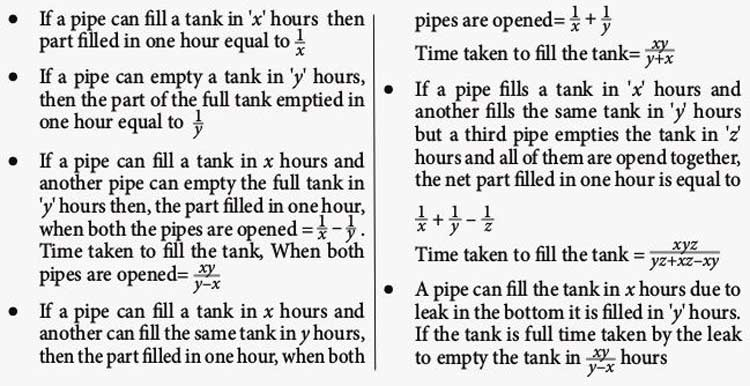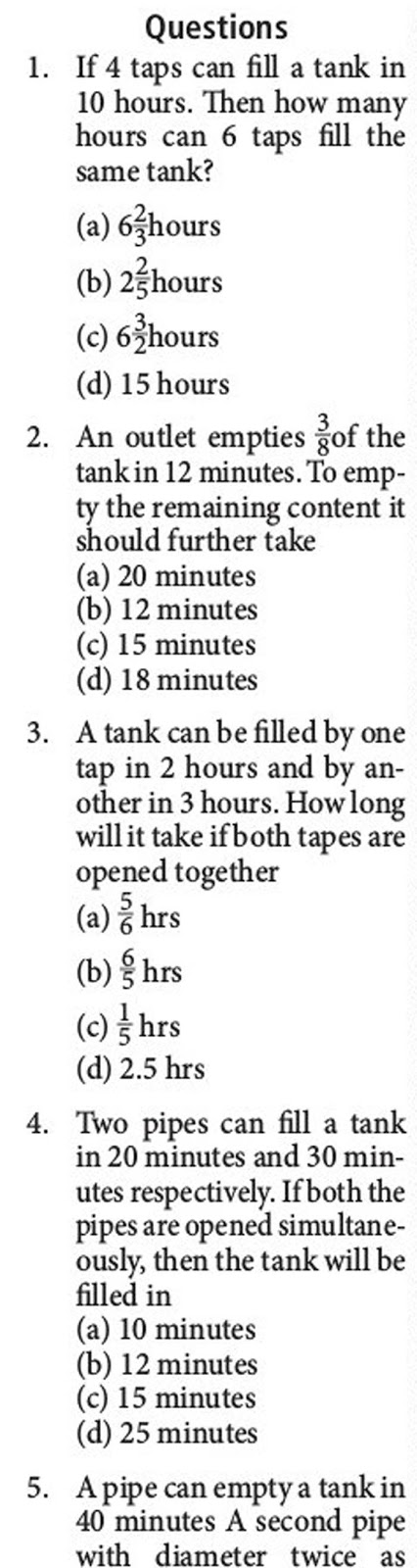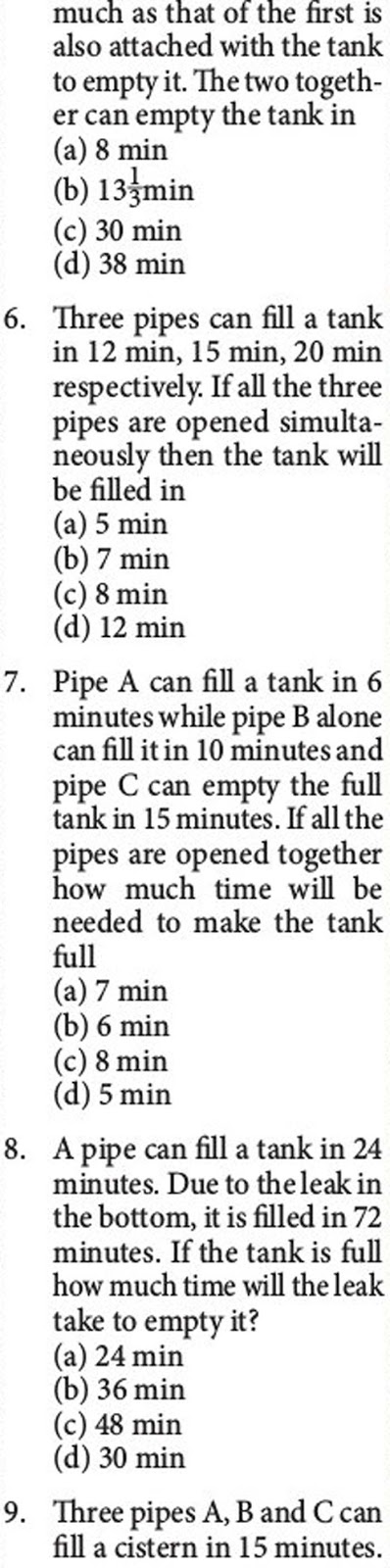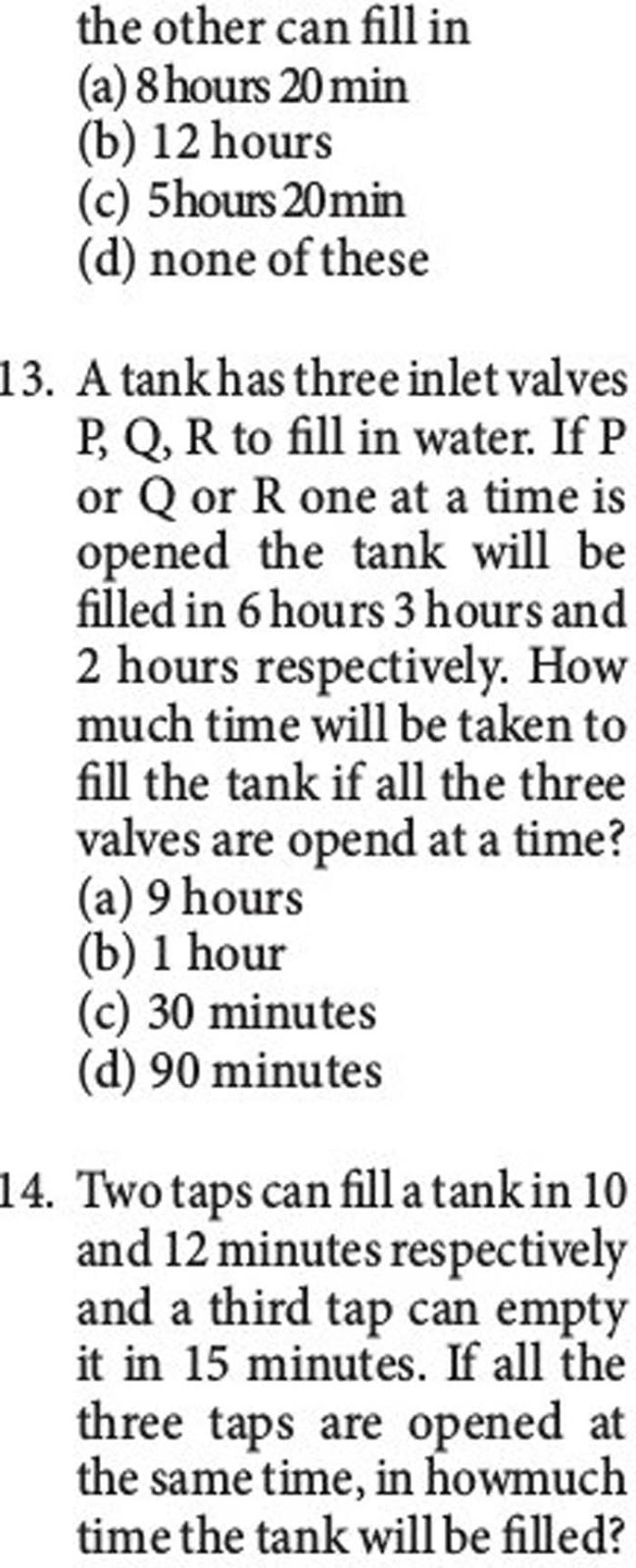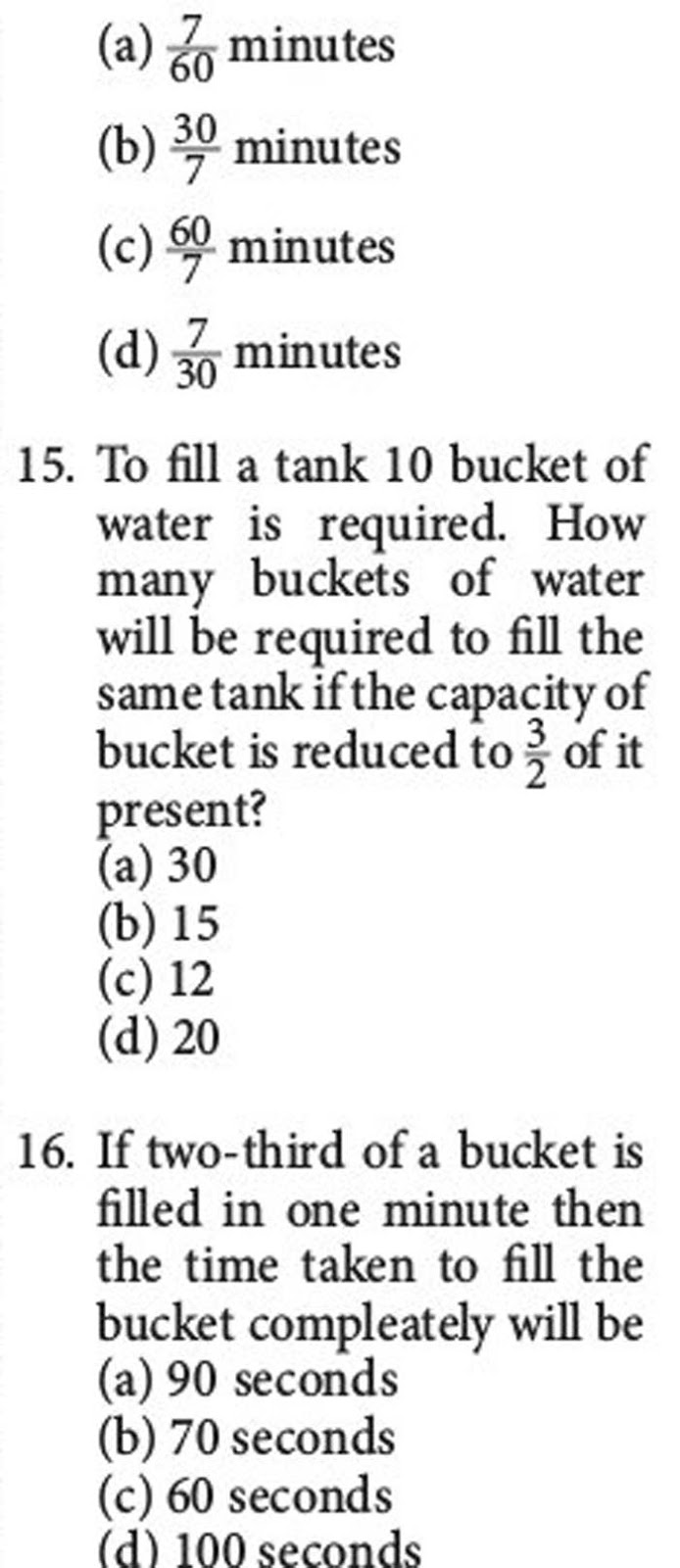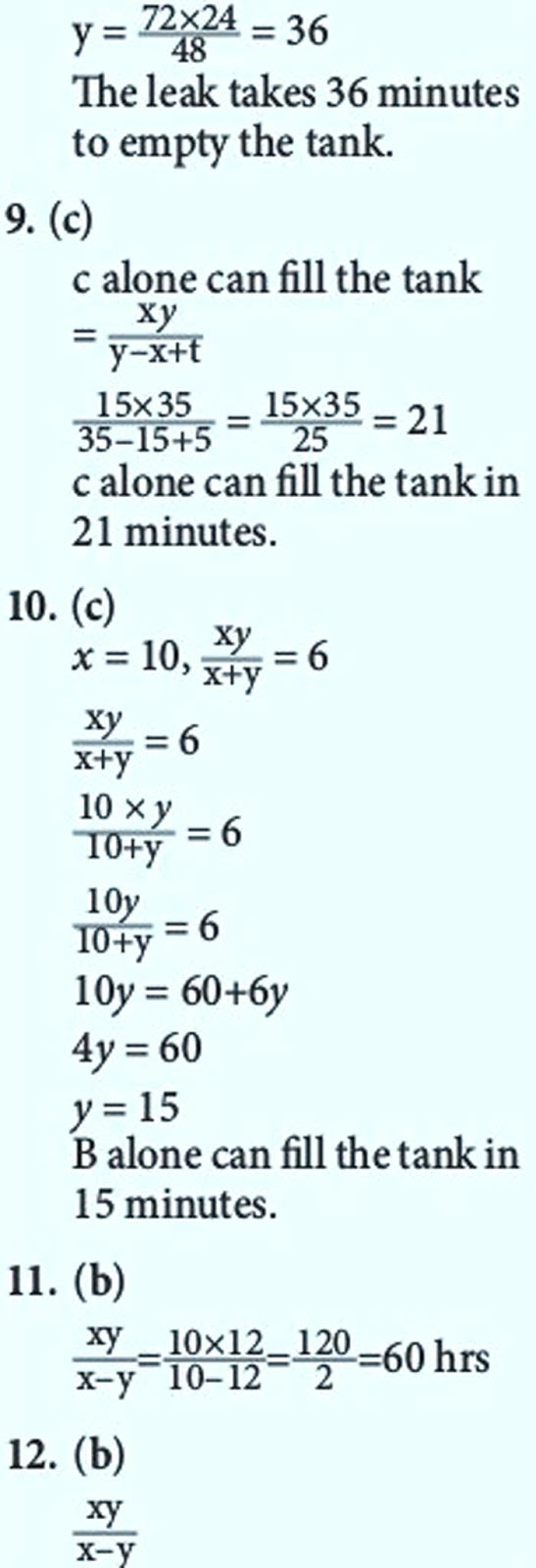* MATHS (SOLVED IN STEPS ==> Ratio and Proportion
* MATHS (SOLVED IN STEPS ==> Progressions
* MATHS (SOLVED IN STEPS ==> Percentage
* MATHS (SOLVED IN STEPS ==> Surds and Indices
* MATHS (SOLVED IN STEPS ==> Calendar
* MATHS (SOLVED IN STEPS ==> Square and cube
* MATHS (SOLVED IN STEPS ==> Simplification
* MATHS (SOLVED IN STEPS ==> Problems on Average
* MATHS (SOLVED IN STEPS ==> HCF & LCM
* MATHS (SOLVED IN STEPS ==> Profit and Loss
* MATHS (SOLVED IN STEPS ==> Problems on Trains
* MATHS (SOLVED IN STEPS ==>
* MATHS (SOLVED IN STEPS ==>
* MATHS (SOLVED IN STEPS ==>
* MATHS (SOLVED IN STEPS ==>